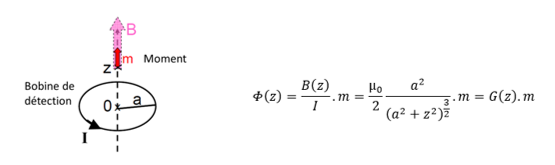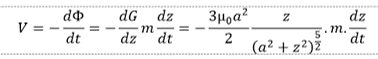Nanomagnetism & Oxides Laboratory
Theory on magnetomretry

Magnetometers are tools dedicated to measure the magnetization of a material. There are composed by a magnetic field source (superconducting coil for studying field up to tense of teslas), a dispositive to vary the temperature (cryostat) and a system to measure the magnetization. The strength or the flux method could be used to do this measurement. In the case of the strength method, the sample is placed inside a homogeneous field. The magnetization is then determined by the measurement of the force that feels the sample. This force is equal to the product of the magnetic moment and of the field gradient. The VSM-SQUID (Quantum Design) is part of the flux method, so we will develop their principle.
The magnetization detection is based on the measurement of the flux ϕ induced by the sample inside a single coil. Thus, a magnetic moment m placed at a height z in the center of a spire (current I, radius a (detective coil)) induces a flux Φ(z) on the spire (Figure 1). The flux can be calculated by using the mutual inductances and the Biot and Savart law.

Figure 1: Schematic of a magnetic moment m placed at a height z in the center of a spire (detective coil of radius a and crossed by a current I) which induce of flux Φ(z) on the spire.

The flux produced by a dipole m on the spire is proportional to its magnetization. Then, the flux could be directly measured. The sample moves periodically, and the voltage at the detective coil bounds is proportional to the induced flux. This voltage could then be measured directly by a voltmeter. Nevertheless, to improve the sensitivity, a SQUID magnetometer (inductively coupled to the detective coil), which induces a voltage proportional to the flux variation, is generally used.
V=Φ(z)=G(z).m
The second possibility is to measure the flux derivative V=dΦ/dt, by varying the sample height z or its moment m thanks to a fluctuating exterior magnetic field. In the VSM mode (Vibrating Sample Magnetometer), the sample vibrates (z(t)) in a constant magnetic field. The voltage is generally measured thanks to a synchronous detection.

Multi-coils configurations, so-called gradiometer, of first order for the VSM (2 spires) and of second order (4 spires) for the SQUID, are generally used to improve the sensitivity. The sensitivity obtained with a SQUID measurement is of the order of 10-10-10-11A.m². This technic is around two orders of magnitude better than for a standard VSM (10-8A.m²). With the VSM-SQUID of Quantum Design, the VSM signal is measured by a SQUID, allowing a sensitivity similar to a standard SQUID.

The origin of uncertunty could be multiple and need to be taken into account especially for sample giving low magnetic signal.
See Garcia et al. JAP, 105, 013925 (2009)
It is first really important, especially for VSM measurement, to correctly centre the sample in the gradiometer.